# Probability - math word problems

Probability is the measure of the likeliness that an event will occur. The probability (chance) is a value from the interval <0;1> or in percentage (0% to 100%) expressing the occurrence of some event. 0 is impossible event and 1 (100%) means the certainty event.

#### Number of problems found: 149

• Probability of failures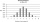In certain productions, the probability of failures is 0.01. Calculate the probability that there will be more than 1 failure among the 100 selected products if we return the selected products to the file after the check.
• Event probability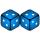The probability of event P in 8 independent experiments is 0.33. What is the probability that the event P occurs in one experiment (chance is the same)?
• Events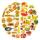Event P has probability of 0.84. What is the probability that the event P occurs in 3, 5, 7 try.
• Double probabilityThe probability of success of the planned action is 60%. What is the probability that success will be achieved at least once if this action is repeated twice?
• Probability of malaria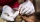A survey carried out at a certain hospital indicates that the probability that a patient tested positive for malaria is 0.6. What is the probability that two patients selected at random (i) one is negative while the other tested positive? (i) both patient
• Shooter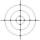The probability that a good shooter hits the center of the target circle I is 0.1. The probability that the target hit intercircle is II 0.58. What is the probability that it hits the target circle I or II?
• Three students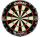Three students independently try to solve the problem. The first student will solve a similar problem with a probability of 0.6, the second student will solve at a probability of 0.55, and the third will solve at a probability 0.04. The problem is resolve
• Probability of intersectionThree students have a probability of 0.7,0.5 and 0.4 to graduated from university respectively. What is the probability that at least one of them will be graduated?
• Three shooters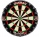Three shooters shoot, each one time, on the same target. The first hit the target with a probability of 0.7; second with a probability of 0.8 and a third with a probability of 0.9. What is the probability to hit the target: a) just once b) at least once c
• Bulbs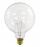The probability that the bulb can operate 4000 hours is 0.3. What is the probability that exactly one of eight bulbs can operate 4000 hours?
• Two doctorsDoctor A will determine the correct diagnosis with a probability of 93% and doctor B with a probability of 79%. Calculate the probability of proper diagnosis if both doctors diagnose the patient.
• Birth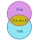Let's assume that the probability of the birth of a boy and a girl in the family is the same. What is the probability that in a family with five children, the youngest and oldest child is a boy?
• DiceWe throw five times the dice. What is the probability that six fits exactly twice?
• Lion or virgin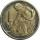We toss coin, every throw fits lion or a virgin with equal probability 1/2. Determine how much at least we have to make throws that with probability 0.9 lion fell at least once.
• FamilyWhat is the probability that a family with 7 childrens have: exactly 5 girls? 7 girls and 0 boys? Consider the birth probability of a girl is 48.69% and boy 51.31%.
• Probability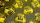What are the chances that the lottery, in which the numbers are drawn 5 of 50 you win the first prize?
• DiceWe throw 10 times a play dice, what is the probability that the six will fall exactly 4 times?
• WordWhat is the probability that a random word composed of chars E, Y, G, E, R, O, M, T will be the GEOMETRY?
• Lottery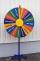The lottery is 60000 elk in which 6200 wins. What is the probability that the purchase of 12 elks won nothing?
• Six on diceWhat is the probability that when throwing two dice will fall at least one six?

Do you have an interesting mathematical word problem that you can't solve it? Submit a math problem, and we can try to solve it.

We will send a solution to your e-mail address. Solved examples are also published here. Please enter the e-mail correctly and check whether you don't have a full mailbox.

Please do not submit problems from current active competitions such as Mathematical Olympiad, correspondence seminars etc...